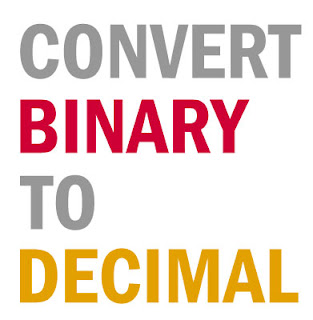## Posts

Showing posts from November, 2019

### How to convert binary number to decimal number using recursion in CRecursive function: int binary(int num,int step){ if(!num){ return num; } printf("step %d: binary no=%d,rem=%d \n",step,num,num%10); num=num%10+2*binary(num/10,step+1); printf("backtrack res step %d (decimal)=%d\n",step,num); return num; } the above function recursively calls itself by passing the binary number whose last digit is removed and stored in num%10 (remainder) which will be added and multiplied by two in each phase while backtracking. num=num%10+2*binary(num/10,step+1); the output of the code along with the values of variables in forward and backward stage are given below: Source Code: // https://www.qxcoding.com/ //binary to decimal include int binary(int num,int step){ if(!num){ return num; } printf("step %d: binary no=%d,rem=%d \n",step,num,num%10); num=num%10+2*binary(num/10,step+1); printf("backtrack res step %